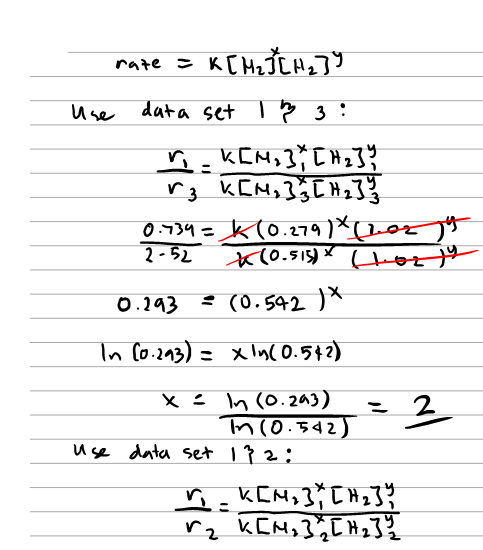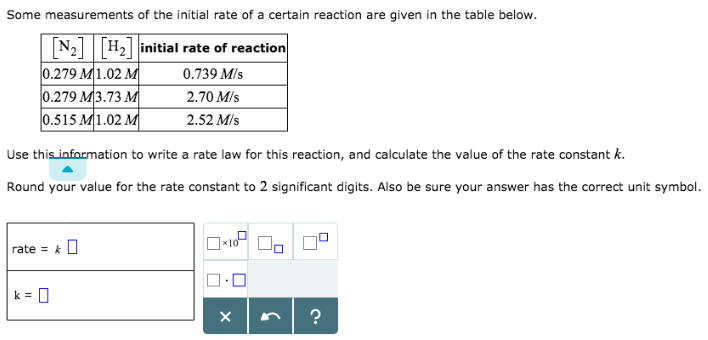# Problem: Some measurements of the initial rate of a certain reaction are given in the table below. Use this information to write a rate law for this reaction, and calculate the value of the rate constant k. Round your value for the rate constant to 2 significant digits. Also be sure your answer has the correct unit symbol.

###### FREE Expert Solution96% (206 ratings)###### Problem Details

Some measurements of the initial rate of a certain reaction are given in the table below.

Use this information to write a rate law for this reaction, and calculate the value of the rate constant k.

Round your value for the rate constant to 2 significant digits. Also be sure your answer has the correct unit symbol.